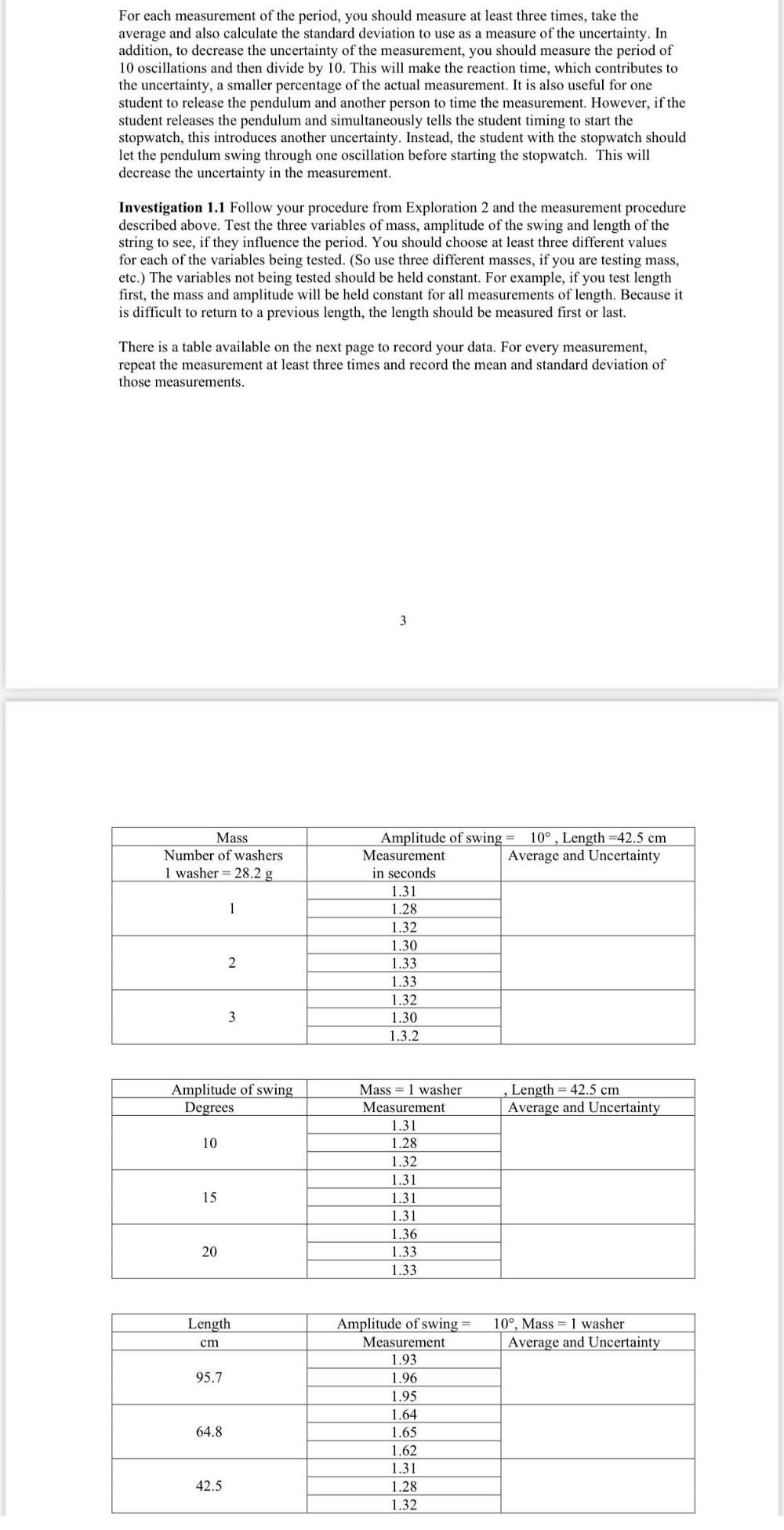Question:

# For each measurement of the period, you should measure at least three times, take the average and also calculate the standard deFor each measurement of the period, you should measure at least three times, take the average and also calculate the standard deviation to use as a measure of the uncertainty. In addition, to decrease the uncertainty of the measurement, you should measure the period of 10 oscillations and then divide by 10. This will make the reaction time, which contributes to the uncertainty, a smaller percentage of the actual measurement. It is also useful for one student to release the pendulum and another person to time the measurement. However, if the student releases the pendulum and simultaneously tells the student timing to start the stopwatch, this introduces another uncertainty. Instead, the student with the stopwatch should let the pendulum swing through one oscillation before starting the stopwatch. This will decrease the uncertainty in the measurement. Investigation 1.1 Follow your procedure from Exploration 2 and the measurement procedure described above. Test the three variables of mass, amplitude of the swing and length of the string to see, if they influence the period. You should choose at least three different values for each of the variables being tested. (So use three different masses, if you are testing mass, etc.) The variables not being tested should be held constant. For example, if you test length first, the mass and amplitude will be held constant for all measurements of length. Because it is difficult to return to a previous length, the length should be measured first or last. There is a table available on the next page to record your data. For every measurement, repeat the measurement at least three times and record the mean and standard deviation of those measurements. 3 Mass Number of washers 1 washer = 28.2 g 1 Amplitude of swing = 10° , Length =42.5 cm Measurement Average and Uncertainty in seconds 1.31 1.28 1.32 1.30 1.33 1.33 1.32 1.30 1.3.2 2 3 Amplitude of swing Degrees Length = 42.5 cm Average and Uncertainty 10 Mass = 1 washer Measurement 1.31 1.28 1.32 1.31 1.31 1.31 1.36 1.33 1.33 15 20 Length 10º, Mass = 1 washer Average and Uncertainty cm 95.7 Amplitude of swing = Measurement 1.93 1.96 1.95 1.64 1.65 1.62 1.31 1.28 1.32 64.8 42.5